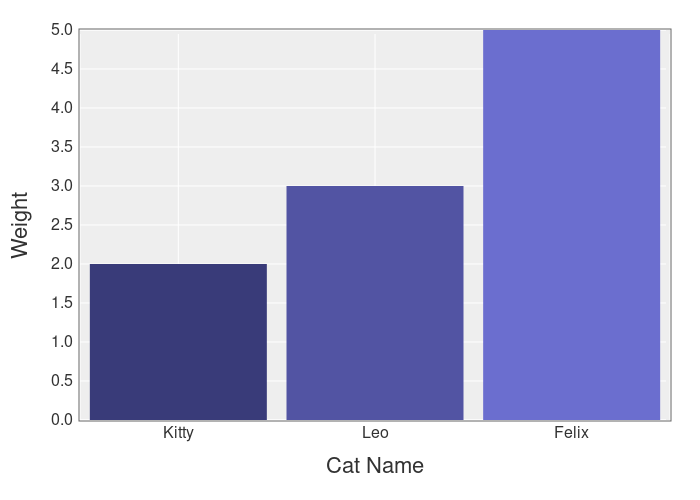# What is A Matrix & How to Use It in Ruby?

A matrix is a 2D (2-dimensional) array that can be used to store & work with spreadsheet-like data.

They can be used for:

• Representing a board in a table game (chess, checkers, etc.)
• Statistics & data analysis
• Generating plots & graphs

Because this is a powerful data structure it’s helpful to learn how to use it.

## How to Create a Matrix in Ruby

You can create a matrix with arrays.

Like this:

```matrix = [
[1,2,3],
[4,5,6],
[7,8,9]
]
```

This produces a 3×3 matrix & it’s your best choice if you want to store 2-dimensional data as a board or set of positions.

But if you would like to combine matrices via addition, subtraction & multiplication…

Then you can use the `Matrix` class.

Here’s how to use it:

```require 'matrix'

a = Matrix[[1,2,3], [4,5,6], [7,8,9]]
b = Matrix[[1,2,3], [4,5,6], [7,8,9]]
```

Now you can add them:

```a + b
# Matrix[[2, 4, 6], [8, 10, 12], [14, 16, 18]]
```

You can access individual values like this:

```a[0, 1]
```

This is different from the array version, which would use this syntax:

```matrix
```

Both array & matrix can be transposed, that means that the rows become columns & the columns become rows.

Example:

```matrix.transpose

# [[1, 4, 7], [2, 5, 8], [3, 6, 9]]
```

Also remember:

Matrix objects are immutable, so you can’t change the values without creating a new matrix. This `Matrix` is mostly for mathematical operations, if you want data analysis & statistics you’ll need something more powerful.

Something like…

## The Daru Gem

Daru is a gem that allows you to work with matrices, get statistics from them & print them as a nicely formatted table. Daru also integrates with Ruby plotting gems so you can generate plots & graphs from your data.

Here’s an example:

```require 'daru'

df = Daru::DataFrame.new(
{
"A" => [1,2,3],
"B" => [4,5,6],
"C" => [7,8,9]
},
index: ["A", "B", "C"]
)
```

This prints the following table:

```    A   B   C
A   1   4   7
B   2   5   8
C   3   6   9
```

You can access a specific column like this:

```df['A']
```

Or by numerical index:

```df
```

And you can get statistics like this:

```df['B'].describe

#         statistics
# count            3
# mean           5.0
# std            1.0
# min              4
# max              6
```

The best part?

You can load data directly from CSV files, ActiveRecord & even Excel files.

Example:

```df = Daru::DataFrame.from_csv('healthy_food.csv')
```

And you can filter the data with a `where` expression.

For example…

If we have a “carbs” column, we can find all the rows in our matrix that have a value of less than 25.

Like this:

```df.where(df['carbs'].lt(25))
```

You can also `sort`, `group_by` & `aggregate` your data frames.

Example:

```df = Daru::DataFrame.new(
{  str: %w(a b c d a),
num: [52,12,7,17,1] }
)

df.group_by(:str).aggregate(num: :sum)

#    num
# a   53
# b   12
# c    7
# d   17
```

## Plotting With Daru

Daru allows you to create visual representations of your data & export them as HTML files.

Here’s an example:

```df = Daru::DataFrame.new(
{'Cat Names' => %w(Kitty Leo Felix),
'Weight'   => [2,3,5]}
)

df.plot(type: :bar, x: 'Cat Names', y: 'Weight') do |plot, _|
plot.x_label 'Cat Name'
plot.y_label 'Weight'

plot.yrange [0, 5]
end
.export_html
```

This produces this chart:You’ll find this chart as an HTML file in the same folder as your code.

If you want to use Daru in your Rails application you’ll need to add the `daru-view` gem to the mix or use a different gem like chartkick.

## Summary

You have learned about matrices in Ruby so you can work with 2-dimensional data!

Don’t forget to share this article so more people can find it.

Thanks for reading.

###### 1 comment
Mitch says a couple of years ago

The daru gem is very interesting to me. Most of my programming is in R. It uses data frames to work with data. I’ve been wanting to learn how to migrate my R scripts to Ruby, so this might help!